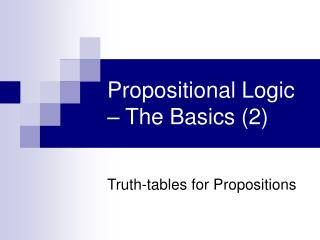DownloadDownload PresentationPropositional Logic – The Basics (2)

# Propositional Logic – The Basics (2)

Télécharger la présentation## Propositional Logic – The Basics (2)

- - - - - - - - - - - - - - - - - - - - - - - - - - - E N D - - - - - - - - - - - - - - - - - - - - - - - - - - -
##### Presentation Transcript

1. Propositional Logic – The Basics (2) Truth-tables for Propositions

2. Assigning Truth True or false? – “This is a class in introductory-level logic.” “This is a class in introductory-level logic, which does not include a study of informal fallacies.” “This is a class in introductory-level logic, which does not include a study of informal fallacies.” L● ~F

3. How about this one? “This is a class in introductory logic, which includes a study of informal fallacies.” “This is a class in introductory logic (T), which includes a study of informal fallacies (F).” L● F TF F

4. Propositional Logic and Truth The truth of a compound proposition is a function of: • The truth value of it’s component, simple propositions, plus • the way its operator(s) defines the relation between those simple propositions. p ● q p v q T F T F F T

5. Truth Table Principles and Rules Truth tables enable you to determine the conditions under which you can accept a particular statement as true or false. Truth tables thus define operators; that is, they set out how each operator affects or changes the value of a statement.

6. Truth and the Actual World Some statements describe the actual world - the existing state of the world at “time x”; the way the world in fact is. “This is a logic class and I am seated in SOCS 203.” - Actually and currently true on a class day. - Possibly true, but not “currently” true on Monday, Wednesday or Friday.

7. Truth and Possible Worlds Some statements describe possible worlds - particular states of the world at “time y”; a way the world could be.. “This is a history class and I am seated in SOCS 203.” Possibly true, but not currently true. Actually true, if you have a history class here and it is a history class day/time. A truth table describes all possible combinations of truth values for a statement. It will, in fact, even tell you if a statement could not possibly be true in any world.

8. Constructing Truth Tables 1. Write your statement in symbolic form. 2. Determine the number of truth-value lines you must have to express all possible conditions under which your compound statement might or might not be true. Method: your table will represent 2n power, where n = the number of propositions symbolized in the statement. 3. Distribute your truth-values across all required lines for each of the symbols (operators will come later). Method: Divide by halves as you move from left to right in assigning values.

9. Constructing Truth Tables - # of Lines For statement forms, there are only two symbols. Thus, these require lines numbering 22 power, or 4 lines.

10. Constructing Truth Tables – Distribution across all Symbols Under “p,” divide the 4 lines by 2. In rows 1 & 2 (1/2 of 4 lines), enter “T.” In rows 3 & 4, (the other ½ of 4 lines), enter “F.” TTFF TTFF

11. Constructing Truth Tables – Distribution across all Symbols Under “q,” divide the 2 “true” lines by 2. In row 1 (1/2 of 2 lines), enter “T.” In row 2, (the other ½ of 2 lines), enter “F.” Repeat for lines 3 & 4, inserting “T” and “F” respectively. TTFF TTFF TF TF TF TF

12. Constructing Truth Tables – Operator Definitions Thinking about the corresponding English expressions for each of the operators, determine which truth value should be assigned for each row in the table. TTFF TTFF T TF TF T FF FFF TF TF T

13. Constructing Truth Tables - # of Lines Remember that you are counting each symbol, not how many times symbols appear. 2 symbols: 1 appearance of “p” and 2 appearances of “q”

14. Exercises - 1 Using the tables which define the operators, determine the values of this statement. ( M > P ) v ( P > M ) TTFF TFTT TFTF TTTT TFTF TTFT TTFF

15. Exercises – 2 Using the tables which define the operators, determine the values of this statement. TTTTFFFF TTFFTTTT TTFFTTFF TTFFTFTF FFFFTTTT TTTTFFFF TTTTTFTF TFTFTFTF FFFFFFFF FFFTFFFT TTFFTTFF TTTFTTTF TFTFTFTF TTFFTTFF